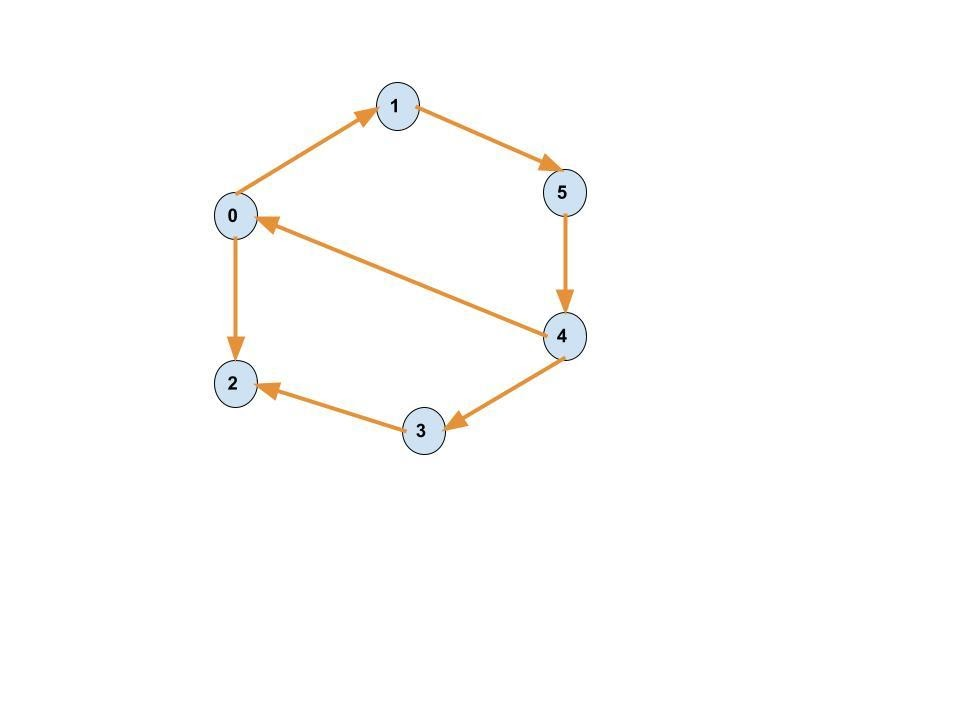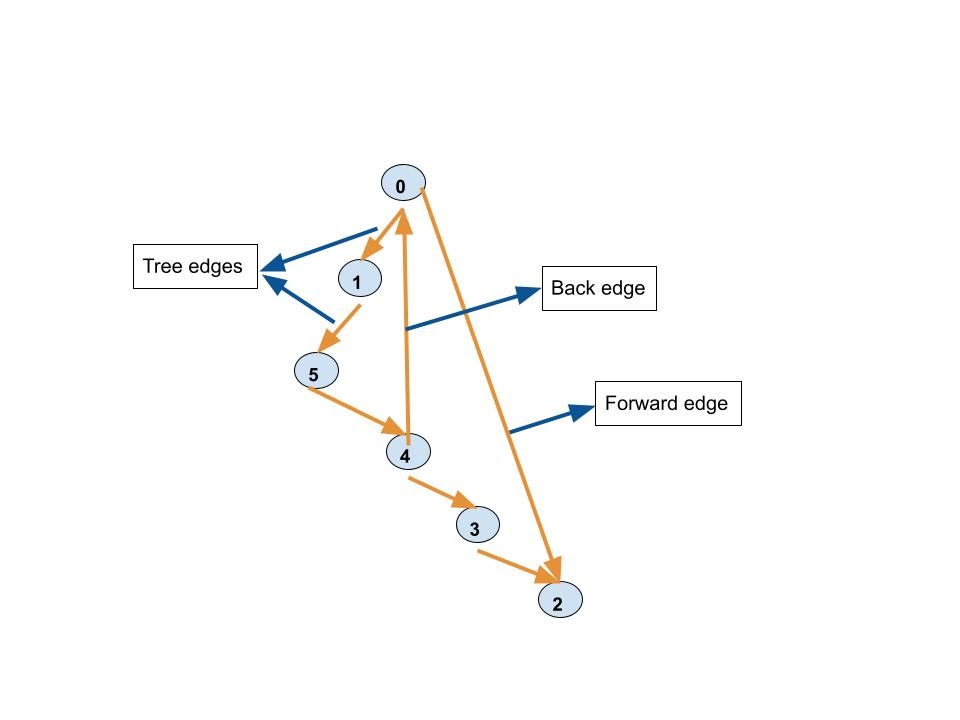# Detect cycles in a directed graph.

## Introduction

Graphs are one of the topics in Data Structures where people tend to struggle more however is not all that complicated once you grasp the basic concepts. Here we are going to discuss one of the most basic concepts that are utilized numerous times, not only just in graphs but at many Medium and Hard difficulty level problems. Let's get right to it.

Suppose you are given a directed graph, and you are asked to check if it contains a cycle or not. To solve this problem, before diving into the solution, let’s first know about the directed graphs and cycles.

A graph whose edges allow only a certain direction of flow is known as a directed graph, or a graph having directed edges and a cycle in a directed graph is a non-empty trail with repeated first and last vertices.For example, the above graph contains the cycle: 1->5->4->0->1

Recommended to try the problem yourself first before moving on to the solution.

## Solution using DFS

This approach takes the help of Depth First Search to detect cycles in a directed graph. We know that the DFS of a directed graph produces a DFS tree, which is a reordered representation of the edges and vertices of the graph.

Now, this tree could have edges of different types, for example, forward edges, tree edges, cross edges, and back edges. So, before moving further, let’s have a brief introduction of these kinds of edges:

• A forward edge is an edge that connects a node to its descendent without being a part of the DFS tree.
• Tree edges are the edges obtained by the DFS traversal of the graph.
• A cross edge is an edge that joins two vertices that don’t have a parent-child relationship among themselves.
• A back edge is an edge that connects a vertex to its ancestor in the DFS tree, and if a DFS tree of a graph has a back edge, it contains a cycle, as the back edge will allow it to have a trail with repeated first and last nodes.

We implement this by using an additional array that contains all the visited vertices and a recursion stack to keep track of the order of appearance of the vertices in the current recursion stack, which ultimately helps in backtracking. Let’s have a look at the DFS tree of the graph:So, we will start with the DFS of the tree and check if we find a back edge in it. If yes, the graph has a cycle, and if not, the graph is acyclic.

## Algorithm

1. Take the number of edges and vertices as user input.
2. Initialize the current index, visited, and recursion stack using recursion
3. Mark the index in the recursion stack and the current node as visited.
4. Make recursive calls (see recursion) for all the unvisited adjacent vertices for the current node, and if any of these recursive functions return true, return true as the final answer.
5. If any adjacent vertices are already visited in the recursion stack, mark the answer as true.
6. Return false if none of the above steps returns true.

### Implementation of the solution

``````#include<bits/stdc++.h>
using namespace std;
//Class for the graph.
class graph
{
int v;
//Function to detectcycle.
bool checkcycle2(int v, bool vertvisited[], bool *recursionst);
public:
graph(int v);
void drawedge(int v, int u);
bool checkcycle();
};
//Constructor for a graph with v nodes.
graph::graph(int v)
{
this->v=v;
}
//To add edges in the graph.
void graph::drawedge(int v, int u)
{
}
//Function to keep track of visited nodes and recursion
//stack.
bool graph::checkcycle2(int v, bool vertvisited[], bool *recursionst)
{
if(vertvisited[v]==false)
{
//Marking the vertex as visited.
vertvisited[v]=true;
recursionst[v]=true;
//Making recursive calls for the adjacent
//vertices and return true if any back edge is
//found.
list<int>::iterator itr;
{
if(!vertvisited[*itr]&&checkcycle2(*itr, vertvisited, recursionst))
{
return true;
}
else if(recursionst[*itr])
{
return true;
}
}
}
//Unmark the vertex from recursion stack.
recursionst[v]=false;
return false;
}
bool graph::checkcycle()
{
//Declare and initialise the visited and recursion stack array as false.
bool *vertvisited=new bool[v];
bool *recursionst=new bool[v];
for(int i=0;i<v;i++)
{
vertvisited[i]=false;
recursionst[i]=false;
}
//Call the "checkcycle2" function for cycle
//detection.
for(int i=0;i<v;i++)
{
if(checkcycle2(i, vertvisited, recursionst))
{
return true;
}
}
return false;
}
//Driver function.
int main()
{
graph g(6);
g.drawedge(0, 1);
g.drawedge(1, 5);
g.drawedge(5, 4);
g.drawedge(4, 0);
g.drawedge(4, 3);
g.drawedge(3, 2);
g.drawedge(0, 2);
//Function call and print the result.
if(g.checkcycle())
cout << "Graph is cyclic";
else
cout << "Graph is acyclic";
return 0;
}``````

Output

``Graph is cyclic``

### Complexity Analysis

Time Complexity: The time complexity of the above approach to detect cycles in a directed graph is O(V+E), where V is the number of vertices in the graph and E is the number of edges. This happens because we are performing the DFS on the tree, which has the same time complexity.

Space Complexity: The space complexity of the above approach is O(V), where V is the number of vertices as we need to maintain a visited set and recursive stack.

### How do you determine if a directed graph has a cycle?

If a directed graph has a back edge in its DFS tree, we can conclude that it contains a cycle.

### What is a cycle in a directed graph?

A cycle in a directed graph is a trail with repeated first and last vertex.

### Can BFS be used to find cycles in directed graphs?

We can use BFS for cycle detection in directed graphs, but it takes more memory than DFS-based methods.

### Is a self-loop a cycle?

Yes, self-loops are considered a cycle in a directed graph because they also have a repeated first and last node.

### What is the eulerian cycle in a graph?

A cycle in a graph that includes each graph edge exactly once is called the eulerian cycle.

## Conclusion

In this blog, we have discussed one of the methods to detect cycles in a directed graph.

The idea is to do a DFS traversal of the graph and maintain an array of visited vertices. During the DFS traversal, if we find a back edge, i.e., an edge that connects back to an already visited verified, we will return the answer that the graph is cyclic. Here we maintained two arrays of vertices, one for keeping track of the visited vertices and the other for the recursion call stack for the DFS traversal.

#### Want to solve more problems? Check these out:

Also, check out some Interview Experiences on Codestudio.

Happy Coding!!